In this post, we are going to explore non-linear data structures like graphs. Also, we’ll cover the central concepts and typical applications.

You are probably using programs with graphs and trees. Let’s say for instance that you want to know the shortest path between your workplace and home, you can use graph algorithms to get the answer! We are going to look into this and other fun challenges.

In the previous post, we explore linear data structures like arrays, linked lists, sets, stacks and so on. This one builds on top of what we learned.

This post is part of a tutorial series:

Learning Data Structures and Algorithms (DSA) for Beginners

Here is the summary of the operations that we are going to cover on this post:

Space *O(|V| + |E|)*
removeVertex *O(|V| + |E|)*
removeEdge (using Array) *O(|E|)*
removeEdge (using HashSet) O(1)

## Graphs Basics

A graph is a data structure where a node can have zero or more adjacent elements.

The connection between two nodes is called edge. Nodes can also be called vertices.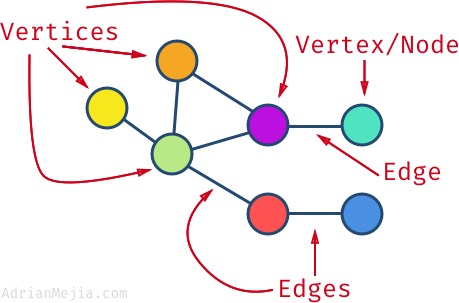The degree is the number of edges connected to a vertex. E.g., the `purple` vertex has a degree of 3 while the `blue` one has a degree of 1.

If the edges are bi-directional, then we have an undirected graph. But, if the edges have a direction, then we have a directed graph or di-graph for short. You can think of it as a one-way street (directed) or two-way street (undirected).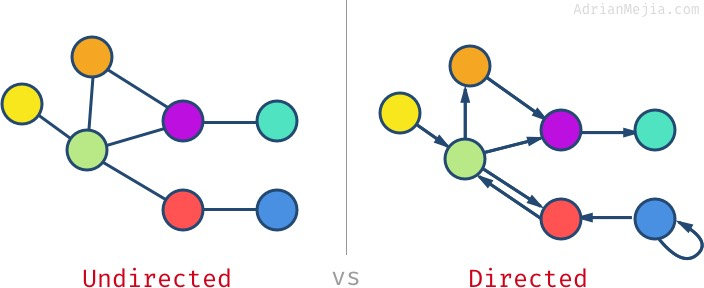Vertex can have edges that go to itself (e.g., `blue` node), this is called self-loop.

A graph can have cycles which means that if you traverse through the node, you could get the same node more than once. The graph without cycles is called acyclic graph.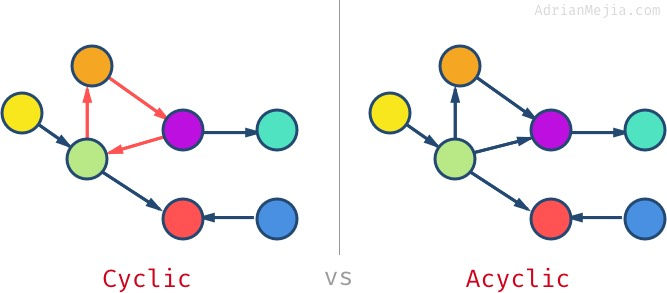Also, acyclic undirected graphs are called tree. We are going to cover trees in depth in the next post.

Not all vertices have to be connected in the graph. You might have isolated nodes or even separated subgraphs. If all nodes have at least one edge, then we have a connected graph. When all nodes are connected to all other nodes, then we have a complete graph.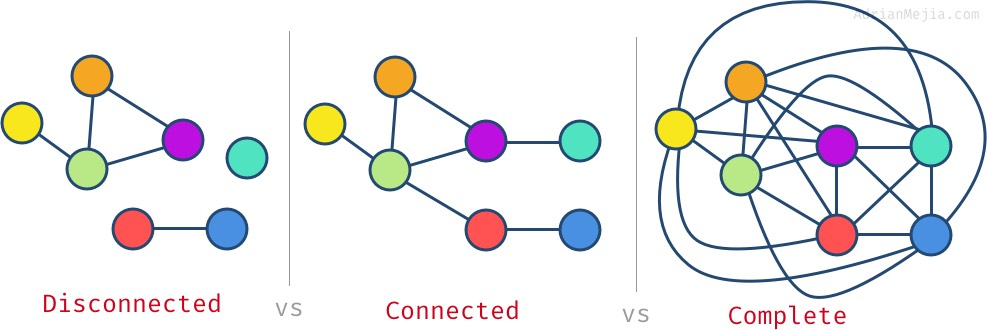For a complete graph, each node should have `#nodes - 1` edges. In the previous example we have 7 vertices, so each node has 6 edges.

## Graph Applications

When edges have values/cost assigned to them, we say we have a weighted graph. If the weight is absent, we can assume it’s 1.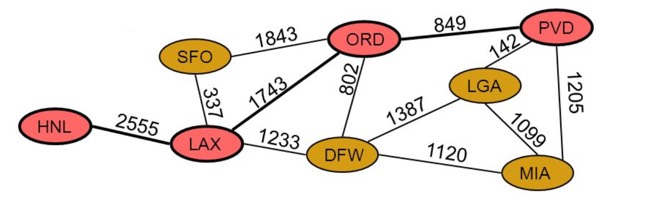Weighted graphs have many applications depending on the domain where you need to solve a problem. To name a few:

• Airline Traffic (image above)

• Node/vertex = Airport
• Edges = direct flights between two airports
• Weight = miles between two airports

• Weight = time required to go from one intersection to another
• Networks routing

• Node = server
• Weight = connection speed

In general, graphs have many real-world applications like:

• Electronic circuits
• Flight reservations
• Driving directions
• Telcom: Cell tower frequency planning
• Social networks. E.g., Facebook uses a graph for suggesting friends
• Recommendations: Amazon/Netflix uses graphs to make suggestions for products/movies
• Graphs help to plan the logistics of delivering goods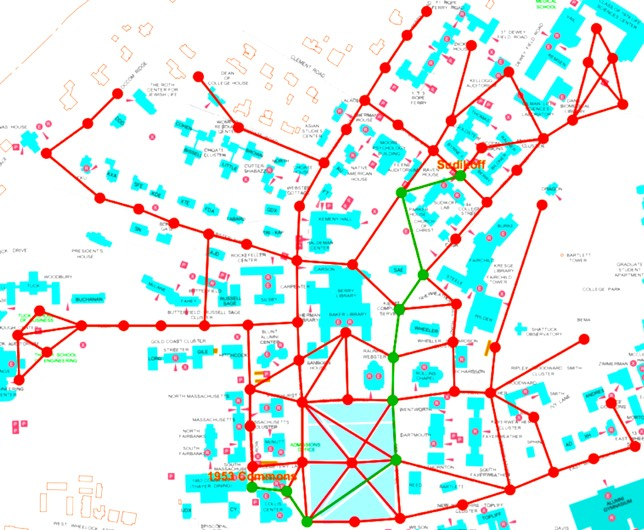We just learned the basics of graphs and some applications. Let’s cover how to represent graphs in JavaScript.

## Representing graphs

There are two primary ways of representing graph:

Let’s explain it with the following directed graph (digraph) as an example: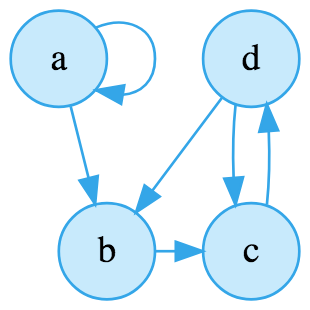We digraph with 4 nodes. When a vertex has a link to itself (e.g. `a`) is called self-loop.

The adjacency matrix is one way of representing a graph using a two-dimensional array (NxN matrix). In the intersection of nodes, we add 1 (or other weight) if they are connected and `0` or `-` if they are not connected.

Using the same example as before, we can build the following adjacency matrix:

As you can see, the matrix list all nodes horizontally and vertically. If there a few connections we called sparse graph if there are many connections (close to the max number of links) we called it dense graph. If all possible connections are reached, then we have a complete graph.

It’s important to notice that for undirected graphs the adjacency matrix will always be symmetrical by the diagonal. However, that’s not always the case on a digraph (like our example).

What is the time complexity of finding connections of two vertices?

Querying if two nodes are connected in an adjacency matrix is O(1).

What is the space complexity?

Storing a graph as an adjacency matrix has a space complexity of O(n2), where `n` is the number of vertices. Also, represented as O(|V|2)

What is the runtime to add a vertex?

The vertices are stored as a `V`x`V` matrix. So, every time a vertex is added, the matrix needs to be reconstructed to a `V+1`x`V+1`.

Since the matrix has a VxV matrix, to get all the adjacent nodes to a given vertex, we would have to go to the node row and get all its edges with the other nodes.

In our previous example, let’s say we want all the adjacent nodes to `b`. We have to get the full row where b is with all the other nodes.

We have to visit all nodes so,

Imagine that you need to represent Facebook network as a graph. You would have to create a matrix of 2 billion x 2 billion, where most of it would be empty! Nobody would know everybody else just a few thousands at most.

In general, we deal with sparse graphs so the matrix will waste a lot of space. That’s why in most implementation we would use an adjacency list rather than the matrix.

Adjacency List is one of the most common ways to represent graphs. Each node has a list of all the nodes connected to it.

Graphs can be represented as an adjacency list using an Array (or HashMap) containing the nodes. Each of these node entries includes a list (array, linked list, set, etc.) that list its adjacent nodes.

For instance, in the graph above we have that `a` has a connection to `b` and also a self-loop to itself. In turn, `b` has a connection to `c` and so on:

As you can imagine if you want to know if a node is connected to another node, you would have to go through the list.

Querying if two nodes are connected in an adjacency list is O(n), where `n` is the number of vertices. Also represented as O(|V|)

Storing a graph as an adjacency list has a space complexity of O(n), where `n` is the sum of vertices and edges. Also, represented as O(|V| + |E|)

## Adjacency List Graph HashMap Implementation

The adjacency list is the most common way of representing graphs. There are several ways to implement the adjacency list:

One of them is using a HashMap. The `key` is the value of the node, and the `value` is an array of adjacency.

Graph usually needs the following operations:

Let’s say that we want to remove the vertex `b`. We could do `delete graph['b'];`, however, we still have to remove the references on the adjacency list on `d` and `a`.

Every time we remove a node, we would have to iterate through all the nodes’ list O(|V| + |E|). Can we do better? We will answer that soon, but first, let’s *implement our list in a more object-oriented way so we can swap implementations easily.

## Adjacency List Graph OO Implementation

Let’s start with the `Node` class that holds the vertex’s value and its adjacent vertices. We can also have helper functions for adding and removing adjacent nodes from the list.

Notice that `adjacent` runtime is O(1), while `remove adjacent` is O(|E|). What if instead of an array use a HashSet 🧐? It could be O(1). But, let first get it working and later we can make it faster.

Make it work. Make it right. Make it faster.

Ok, now that we have the `Node` class, let’s build the Graph class that can perform operations such as adding/removing vertices and edges.

Graph.constructor

The first thing that we need to know is if the graph is directed or undirected. That makes a difference when we are adding edges.

To add an edge we need two nodes. One is the source, and the other is the destination.

We add an edge from the source vertex to the destination. If we have an undirected graph, then we also add from target node to source since it’s bidirectional.

The runtime of adding an edge from a graph adjacency list is: O(1)

If we try to add an edge and the nodes don’t exist, we need to create them first. Let’s do that next!

The way we create a node is that we add it to the `this.nodes` Map. The map store a key/value pair, where the `key` is the vertex’s value while the map `value` is the instance of the node class. Take a look at line 5-6:

If the node already exists we don’t want to overwrite it. So, we first check if it already exists and if it doesn’t, then we create it.

The runtime of adding a vertex from a graph adjacency list is: O(1)

Graph.removeVertex

Removing a node from the graph, it’s a little bit more involved. We have to check if the node to be deleted it’s in use as an adjacent node.

We have to go through each vertex and then each adjacent node (edges).

The runtime of removing a vertex from a graph adjacency list is O(|V| + |E|)

Finally, let’s remove implement removing an edge!

Graph.removeEdge

Removing an edge is pretty straightforward and similar to `addEdge`.

The main difference between `addEdge` and `removeEdge` is that:

• If the vertices don’t exist, we won’t create them.
• We use `Node.removeAdjacent` instead of `Node.addAdjacent`.

Since `removeAdjacent` has to go through all the adjacent vertices we have the following runtime:

The runtime of removing an edge from a graph adjacency list is O(|E|)

We are going to explore how to search for values from a node.

Breadth-first search is a way to navigate a graph from an initial vertex by visiting all the adjacent nodes first.Let’s see how we can accomplish this in code:

As you can see, we are using a `Queue` where the first node in is also the first node to be visited (FIFO).

We are as well using JavaScript generators, notice the `*` in front of the function. This generator iterates one value at a time. That’s useful for large graphs (millions of nodes) because in most cases you don’t need to visit every single node.

This an example of how to use the BFS that we just created:

You can find more illustrstions of usage in the test cases. Let’s move on to the DFS!

Depth-first search is another way to navigate a graph from an initial vertex by recursively the first adjacent node of each vertex found.The iterative implementation of a DFS is identical to the BFS, but instead of using a `Queue` you use a `Stack`:

We can test our graph as follow.

As you can see the graph is the same on BFS and DFS, however, the order how the nodes were visited is very different. BFS went from 1 to 10 in that order, while DFS went as deep as it could on each node.

## Graph Time and Space Complexity

We have seen some of the basic operations of a Graph. How to add and remove vertices and edges. Here’s a summary of what we have covered so far: